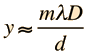# Diffraction Grating

A diffraction grating is the tool of choice for separating the colors in incident light.The condition for maximum intensity is the same as that for a double slit. However, angular separation of the maxima is generally much greater because the slit spacing is so small for a diffraction grating.

Displacement y = (Order m x Wavelength x Distance D)/(slit separation d)

For a diffraction grating with lines/mm = lines/inch,

the slit separation is d = micrometers = x10^ m.

 For incident light wavelength λ = nm at order m =,

projected on a screen at distance D = cm,

the displacement from the centerline for maximum intensity will be= cm.

 This corresponds to an angle of θ = ° .

The resolvance of such a grating depends upon how many slits are actually covered by the incident light source; i.e., if you can cover more slits, you get a higher resolution in the projected spectrum.
If N = slits are illuminated, then the resolvance R = .

This resolvance implies that the wavelength resolution is

 Δλ = nm
 which corresponds to a spacing of Δy = cm at the screen position.

This calculation is designed to allow you to enter data and then click on the quantity you wish to calculate in the active formula above. The data will not be forced to be consistent until you click on a quantity to calculate. Default values will be entered for unspecified parameters, but all values may be changed.

Note: The small angle approximation was not used in the calculations above, but it may be sufficiently accurate for laboratory calculations.

 Discussion Illustration

Index

Grating concepts

 HyperPhysics***** Light and Vision R Nave
Go Back